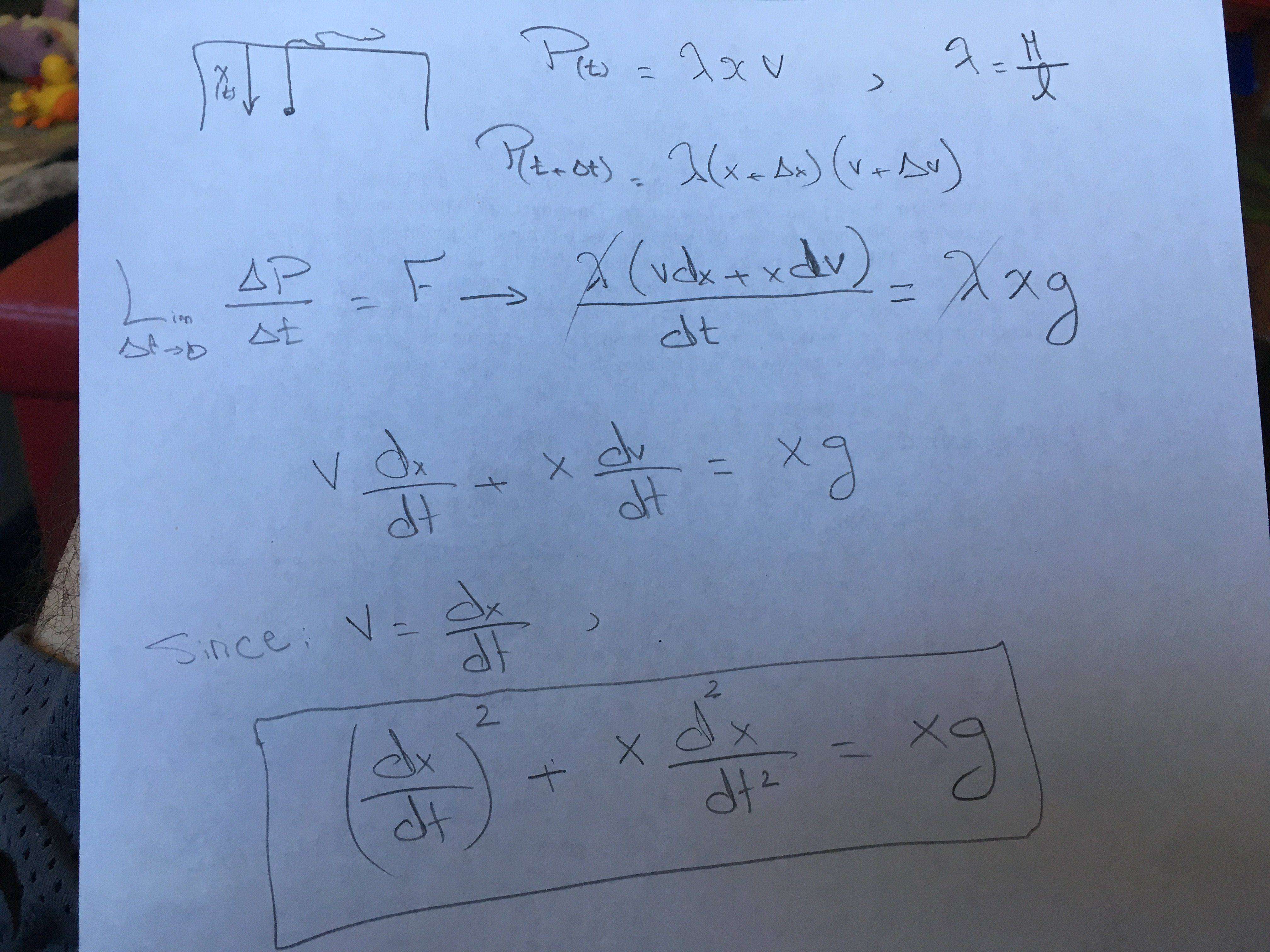# Rope on Table Kleppner-Mechanics

## Homework Statement

A rope of mass M and length l lies on a frictionless table, with a short portion, l0 hanging through a hole. Initially the rope is at rest. a. Find a general solution for x(t), the length of rope through the hole.

## The Attempt at a Solution

Solution uploaded. Where did I go wrong! My differential equation is different than Kleppner's.
Thanks[/B]

#### Attachments

BvU
Homework Helper
What is P ?

What is P ?
Linear Momentum going down positive

BvU
Homework Helper
That the only thing that moves ?

That the only thing that moves ?
Rope on table moving along horizontally not down so yes
I am summing momentum along the vertical direction

BvU
Homework Helper
So where does the motion of the horizontal part of the rope come from? Spontaneity ?

haruspex
Homework Helper
Gold Member
A rope of mass M and length l lies on a frictionless table
It is unclear whether it lies in a formless heap or in a straight line. If in a line then it is unclear exactly how it passes through the hole. You have assumed a heap. Can you find a different assumption which matches the book equation?

I am still not sure from replies either way what difference it makes?
are you suggesting there is also Tension Up on top of the moving piece? needs factored to the force? would it not be zero anyways if we consider the nonmoving part of the rope ie no forces there HORIZANTALLY so T = 0 ? and no friction so rope should slide towards hole freely?

haruspex
Homework Helper
Gold Member
the nonmoving part of the rope
If the portion on the table is straight then there are no nonmoving (indeed, no non-accelerating) parts.

If the portion on the table is straight then there are no nonmoving (indeed, no non-accelerating) parts.
Thank you. I see. You are correct. But now the question is how do I find ∫ F dt between t and t+dt

haruspex
Homework Helper
Gold Member
Thank you. I see. You are correct. But now the question is how do I find ∫ F dt between t and t+dt
As I mentioned in post #7, if the tabletop portion is straight there is still a question mark over exactly how it passes through the hole.
Most likely, the question setter assumes work is conserved. E.g. imagine a hole in the form of a smooth tube bent into an arc, horizontal at the top and vertical below. In this way, the horizontal momentum of the rope across the table gets converted into vertical momentum without KE loss.
At the other extreme, as each dx of rope reaches the top of the hole it effectively slams into a wall, losing all its momentum and KE.
These scenarios will lead to different ODEs.

As I mentioned in post #7, if the tabletop portion is straight there is still a question mark over exactly how it passes through the hole.
Most likely, the question setter assumes work is conserved. E.g. imagine a hole in the form of a smooth tube bent into an arc, horizontal at the top and vertical below. In this way, the horizontal momentum of the rope across the table gets converted into vertical momentum without KE loss.
At the other extreme, as each dx of rope reaches the top of the hole it effectively slams into a wall, losing all its momentum and KE.
These scenarios will lead to different ODEs.
Got it. Ok thanks so much.